## Monday 26 March 2018

### Simple Quantum Chemistry: Hartree-Fock in Python

HF-Copy1

Computational chemistry allow properties of molecules to be determined with incredible accuracy. This includes how much energy is stored in a molecule (and how much is released when you combust it), at which frequencies does it vibrate (which can be used to identify chemicals), how quickly a reaction will occur etc. . One of the most widely used method to calculate these properties as a first approximation is the Hartree-Fock (HF) method. Many very successful widely used density functional methods (B3LYP, PBE0, B97) will add in exact exhange calculated using HF to improve the calculation. One of the classic texts in computational chemistry is Modern Quantum Chemistry by Szabo and Ostlund. The book includes a simple calculation of the molecule $HeH^+$ which has the same electronic configuration as the hydrogen molecule but due to the +2 charge on the helium nucleus it is not symmetrical and must be calculated iteratively by converging on a consistent set of parameters which is why the method is often called self consistent field theory (SCF). I have rewritten this example in the python language based on the original Fortran code and try to explain how the calculations are performed and why the operations are done. I have also added in figures to show what the results look like.

## Molecular Schrodinger equation¶

We begin with the time-independent Schrodinger equation for a molecule is

$$H\Psi = E\Psi$$

The Hamiltonian $H$ performs some mathematical operations on the function $\Psi$ and returns a number the energy and the $\Psi$ function unchanged. This equation is what is called a eigenvalue equation and turns out to be fairly straightforward to solve particularly if you make use of matrix algebra which is the method used in with the Roothmaan-Hall equations.

$$\left[ -\sum_i \frac{\nabla_i^{2}}{2} -\sum_A \frac{\nabla_A^{2}}{2} - \sum_{A,i}\frac{Z_{A}}{r_{Ai}}+\sum_{A>B}\frac{Z_A Z_B}{R_{AB}}+\sum_{i>j}\frac{1}{r_{ij}}\right]\Psi(\boldsymbol{r};\boldsymbol{R})=E\Psi(\boldsymbol{r};\boldsymbol{R})$$

or in a more compact form

$$\left[\hat{T}_e(\boldsymbol{r}) +\hat{T}_N(\boldsymbol{R}) +\hat{V} _{eN}(\boldsymbol{r};\boldsymbol{R})+\hat{V}_{NN}(\boldsymbol{R})+\hat{V}_{ee}(\boldsymbol{r})\right]\Psi(\boldsymbol{r};\boldsymbol{R})=E\Psi(\boldsymbol{r};\boldsymbol{R})$$

You will notice that there are no units because we are making use of atomic units (a.u.) which simplifies the equations by equating these constants to one $m_e=e=h/2\pi=1/(4\pi\epsilon_{0})=1$.

The sums $\Sigma$ have electron $i,j$ and nuclei $A,B$ indices. With the electron positions $\boldsymbol{r}$ and nuclei position $\boldsymbol{R}$ being used to calculate distances between nuclei-electrons $r_{Ai}$, nuclei-nuclei $r_{AB}$ and electron-electron $r_{ij}$. Atomic charges are needed $Z_{A/B}$ in our case $Z_{He}=+2$ and $Z_{H}=+1$.

The terms are, in order;

• Sum of each electron's kinetic energy $$\hat{T}_e(\boldsymbol{r})=-\sum_i \frac{\nabla_i^{2}}{2}$$
• Using classical mechanics we can determine the kinetic energy from the velocity $\boldsymbol{v}$ and mass $m$ of the particle (multiplied together to give the momentum $\boldsymbol{p}=m\boldsymbol{v}$) $$E_k=T=\frac{1}{2}m\boldsymbol{v}^2=\frac{\boldsymbol{p}^2}{2m}$$.
• This classical definition cannot be applied in quantum mechanics as you cannot define a definite position $\boldsymbol{r}$ due to the uncertainity principle which states that the momentum and position cannot be known to any degree of accuracy.
• We must make use of the quantum mechanical momentum operator $\hat{p} =-i\hbar\nabla$ which comes from the wave description of a free particle. $$T_e = \frac{\boldsymbol{p}^2}{2m} = \frac{(-i\hbar\nabla)^2}{2m} = -\frac{\hbar^2}{2m}\nabla^2$$ ($\nabla$ is the symbol used to specify derivatives in multivariable calculus. i.e $\partial /\partial x$)
• Think of this as a kinetic energy pressure which due to quantum uncertainity holds the electron away from the nuclei allowing it not to collapse to the positive nuclei.
• This fluctuation is dependent inversely on the mass so the fluctuation in the light electron is much larger than the heavy nuclei which means it is only neccessary (in most cases) to consider the qunatum mechanical fluctutations of the electrons around fixed nuclei.
• Sum of each nuclei's kinetic energy $$\hat{T}_N(\boldsymbol{R})=-\sum_A \frac{\nabla_A^{2}}{2}$$
• Just as the electron does not have a definitive position so also the nuclei do not.
• The uncertainity of the electron is much greater than the uncertainty of the nuclei due to the much greater mass of the later that we often will ignore the quantum uncertainity in the nuclei.
• However, for light nuclei such as hydrogen this is important and can lead to interesting quantum tunneling effects.
• Sum of energy from nuclei-electron attraction $$\hat{V} _{eN}(\boldsymbol{r};\boldsymbol{R})= - \sum_{A,i}\frac{Z_{A}}{r_{Ai}}$$
• this is an attractive energy between nuclei and electrons and is just coulombs law which is usually written $E_{Coulomb} = \frac{q_A q_B}{4\pi \epsilon_0R_{AB}}$ with two charge $q_A$ and $q_B$ a distance apart $R_{AB}$
• remember atomic units removes the $4\pi\epsilon_0$ constants, where $\epsilon_0$ is the electric permittivity.
• Sum of energy from nuclei-nuclei repulsion $$\hat{V}_{NN}(\boldsymbol{R})=+\sum_{A>B}\frac{Z_A Z_B}{R_{AB}}$$
• a repulsive term this is just coulombs law usually written $E_{Coulomb} = \frac{q_A q_B}{4\pi \epsilon_0R_{AB}}$ with two nuclear charges $q_A$ and $q_B$ a distance $R_{AB}$ apart
• Sum of electron-electron repulsion $$\hat{V}_{ee}(\boldsymbol{r})=+\sum_{i>j}\frac{1}{r_{ij}}$$
• electron-electron repulsion similar to the electrostatic term between the nuclei and electrons.

Most often, however, we only consider the electronic problem for a given set of nuclear positions $\boldsymbol{R}$ as the electrons are significantly lighter and therefore can be considered to instantanously adjust for any nuclear position. (However, the energy here does not include the nuclear repulsions which need to be added at the end to get the total energy.)

$$\left[ \hat{T}_e(\boldsymbol{r})+ \hat{V} _{eN}(\boldsymbol{r};\boldsymbol{R})+\hat{V}_{ee}(\boldsymbol{r})\right]\Psi(\boldsymbol{r})=E_{el}\Psi(\boldsymbol{r})$$

## Hartree-Fock equation¶

One of the challenges in solving this equation is that you do not know where the electron resides only where it is statisically likely to be if you took a measurement - called the many-body effect. This makes the terms involving the electron repulsions ($+\sum_{i>j}\frac{1}{r_{ij}}$) difficult to calculate.

In the Hartree-Fock approach the first approximation used is to treat each electron separately interacting with an averaged distribution of the other electrons (mean field approach).

$$\left[ -\sum_i \frac{\nabla_i^{2}}{2}- \sum_{A,i}\frac{Z_{A}}{r_{Ai}}+v_{HF}(i)\right]\Psi(\boldsymbol{r})=\epsilon_{ij}\Psi(\boldsymbol{r})$$

The functions chosen to represent each electron is based on the hydrogen atomic solutions, which are exact, for an electron around an atom of hydrogen the atomic orbitals.

So we consider a product of one-electron wavefunctions $\psi_i(\boldsymbol{r})$ interacting with a mean field of all the other electrons. $$\Psi(\boldsymbol{r}) = \psi_1(\boldsymbol{r}_1)\psi_2(\boldsymbol{r}_2)\psi_3(\boldsymbol{r}_3)...=\prod_i \psi_i(\boldsymbol{r}_i)$$

However this does not have the correct symmetry requirements which means if you swap electrons the sign of the wavefunction inverts. This requirement means that the Fermions (electrons) cannot occupy the same quantum states and do not collapse towards the nucleus. We can construct something called a slater determinant which does have the correct symmetry properties.

$$\Psi(\mathbf{r}_1, \mathbf{r}_2, \ldots, \mathbf{r}_N) = \frac{1}{\sqrt{N!}} \left| \begin{matrix} \psi_1(\mathbf{r}_1) & \psi_2(\mathbf{r}_1) & \cdots & \psi_N(\mathbf{r}_1) \\ \psi_1(\mathbf{r}_2) & \psi_2(\mathbf{r}_2) & \cdots & \psi_N(\mathbf{r}_2) \\ \vdots & \vdots & \ddots & \vdots \\ \psi_1(\mathbf{r}_N) & \psi_2(\mathbf{r}_N) & \cdots & \psi_N(\mathbf{r}_N) \end{matrix} \right|\equiv \left|\psi _1,\psi _2,\cdots ,\psi _N\right.\rangle$$

$\left|\psi _1,\psi _2,\cdots ,\psi _N\right.\rangle$ is often written simply as $\psi_i(\boldsymbol{r}_i)$

This means we can write a set of $i$ equations which can be solved for a set of one-electron wavefunctions.

$$\left[ -\sum_i \frac{\nabla_i^{2}}{2}- \sum_{A,i}\frac{Z_{A}}{r_{Ai}}+v_{HF}(i)\right]\psi_i(\boldsymbol{r}_i)=\epsilon_{ij}\psi_i(\boldsymbol{r}_i)$$

What then is $v_{HF}(i)$ we can consider two interactions a coulomb interaction $\mathscr{J}$ (repulsion between the "averaged out" one-electron orbitals) and an exchange interactions $\mathscr{K}$ which is not classical and is due to electrons of the same spin being indistinguishable which lowers the energy of the system.

$$v_{HF}(i) = \sum_j \left[ 2\mathscr{J}_j - \mathscr{K}_j\right]$$

Where the sum is over all of the other $j$ electrons. There is a double contribution from the coulomb operator as we are considering closed shell systems where there are two electrons per orbital but the exhange operator is only considered once as this interaction can only be between electrons with like-spins. This means for our HeH+ system it will not contribute as we have

$$\mathscr{J}_j = \int \psi_j(\boldsymbol{r}_j)^* \frac{1}{r_{i,j}}\psi_j(\boldsymbol{r}_j) dr$$$$\mathscr{K}_j\psi_i(\boldsymbol{r}_i) = \left[\int \psi_j(\boldsymbol{r}_j)^* \frac{1}{r_{i,j}}\psi_i(\boldsymbol{r}_i)dr\right]\psi_j(\boldsymbol{r}_j)$$

You will notice the indices have switched for the exchange operator.

The first two terms are often considered as the core terms as they are the kinetic energy and potential energy for an isolated system such as the hydrogen atom and therefore they are refered to as $\mathscr{H}^{CORE}$

$$\mathscr{H}^{CORE} = -\sum_i \frac{\nabla_i^{2}}{2}- \sum_{A,i}\frac{Z_{A}}{r_{Ai}}$$

Putting this all together we get the Hartree-Fock equation

$$\left( \mathscr{H}^{CORE} + \sum_j \left[ 2\mathscr{J}_j - \mathscr{K}_j\right] \right)\psi_i(\boldsymbol{r}_i) = \sum_j \epsilon_{ij}\psi_i(\boldsymbol{r}_i)$$

Or simply

$$\mathscr{f}_i\psi_i(\boldsymbol{r}_i) = \epsilon_{i}\psi_i(\boldsymbol{r}_i)$$

where $\mathscr{f}_i$ are the fock operators

## Orbitals¶

The most successful method to construct these one-electron wavefunctions $\psi_i$ is to consider them delocalised over the whole molecule. Therefore there will be a set of orbitals, one for each electron, which are spread over the whole molecule. We call this type of treatment molecular orbital theory. The question then is what functions do we use to treat these one-electron delocalised orbitals. We know the solutions exactly for a hydrogen like atom so we can use atomic orbitals $\phi_i(\boldsymbol{r}_i)$ centred at each atom $\boldsymbol{r}_i$ to be the basis for our delocalised molecular orbitals. We need to be able to optimise the amounts of each of these atomic orbitals in each of our one-electron delocalised orbitals so we consider a linear combination of atomic orbitals (LCAO).

$$\psi_i(\boldsymbol{r}_i) = \sum_{\mu} c_{\mu} \phi_{\mu}(\boldsymbol{r}_i)$$

where the constants $c_i$ are going to be optimised to minimise the energy.

The method relies on the vartionial principle which is true for the Hartree-Fock equations which is that any approximation you make (i.e. describing the electrons as a LCAO) will raise the energy above the real energy of the system. The advantage of a variational method is that you can systematically improve the wavefunction and improve your result knowing that you will be able to converge upon a value (which will be above the actual energy).

The atomic orbitals are called the slater type orbitals for the 1s orbital this can be written as

$$\phi^{SLA}\left( \boldsymbol{r}\right) = \left( \zeta^3/\pi \right)^{1/2}e^{-\zeta \boldsymbol{r}}$$

Let's have a look at the function by plotting it.

In :
# Need to import some libraries to have the maths functions and plotting
import numpy as np
import scipy.special as sp
import matplotlib.pyplot as plt
%matplotlib notebook # Allows plotting in the notebook

x = np.linspace(-5,5,num=1000)
r = abs(x)

zeta = 1.0

psi_STO = (zeta**3/np.pi)**(0.5)*np.exp(-zeta*r)

plt.figure(figsize=(4,3))
plt.plot(x,psi_STO)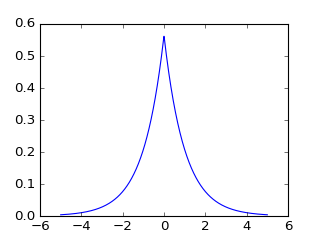Out:
[<matplotlib.lines.Line2D at 0x1065af0b8>]

Slater type orbitals (STO) are the exact solutions for the hydrogen atom and provide an accurate basis set for many electron molecules however the calculations of the integrals are expensive as their is no simple exact solution for the integrals. One way around this is to approximate the Slater type orbitals using a sum of contracted Gaussian functions (CGF). There are simple analytical expressions for the integrals between two Gaussians so this can save a lot of computing time. Let's look at this for the case of the 1s orbital

$$\phi^{GF}(\alpha)=(2\alpha/\pi)^{3/4}exp(-\alpha r^{2})$$$$\phi^{CGF}\left( \boldsymbol{r}\right) = \sum_n d_n\phi^{GF}_n(\alpha)$$

We will make use of three Gaussians to approximate the slater type orbitals.

$$\phi^{CGF}_{STO-3G}\left( \boldsymbol{r}\right) = \sum^3_n d_n\phi^{GF}_n(\alpha)$$
In :
# Coeff is the d_n variable in the equation above
Coeff = np.array([[1.00000,0.0000000,0.000000],
[0.678914,0.430129,0.000000],
[0.444635,0.535328,0.154329]])

# Expon is the alpha variable in the equation above
Expon = np.array([[0.270950,0.000000,0.000000],
[0.151623,0.851819,0.000000],
[0.109818,0.405771,2.227660]])

psi_CGF_STO1G = Coeff[0,0]*(2*Expon[0,0]/np.pi)**(0.75)*np.exp(-Expon[0,0]*r**2)
psi_CGF_STO2G = Coeff[1,0]*(2*Expon[1,0]/np.pi)**(0.75)*np.exp(-Expon[1,0]*r**2) \
+ Coeff[1,1]*(2*Expon[1,1]/np.pi)**(0.75)*np.exp(-Expon[1,1]*r**2) \
+ Coeff[1,2]*(2*Expon[1,2]/np.pi)**(0.75)*np.exp(-Expon[1,2]*r**2)
psi_CGF_STO3G = Coeff[2,0]*(2*Expon[2,0]/np.pi)**(0.75)*np.exp(-Expon[2,0]*r**2) \
+ Coeff[2,1]*(2*Expon[2,1]/np.pi)**(0.75)*np.exp(-Expon[2,1]*r**2) \
+ Coeff[2,2]*(2*Expon[2,2]/np.pi)**(0.75)*np.exp(-Expon[2,2]*r**2)

# Plot the three functions
plt.figure(figsize=(5,3))
plt.title("Approximations to a STO with CGF")
plt.plot(x,psi_STO,label="STO")
plt.plot(x,psi_CGF_STO1G,label="STO-1G")
plt.legend()
plt.figure(figsize=(5,3))
plt.plot(x,psi_STO,label="STO")
plt.plot(x,psi_CGF_STO2G,label="STO-2G")
plt.legend()
plt.figure(figsize=(5,3))
plt.plot(x,psi_STO,label="STO")
plt.plot(x,psi_CGF_STO3G,label="STO-3G")
plt.legend()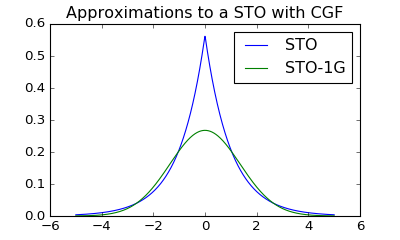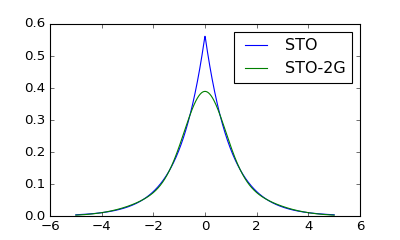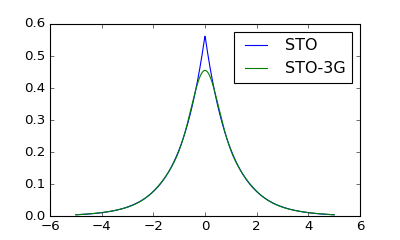Out:
<matplotlib.legend.Legend at 0x106ddda58>

As the number of Gaussians is increased the function more closely describes the slater type orbitals. You will also see that nearest the centre (x=0) the approximation is poorest. This region is called the cusp.

We will use this very poor basis set for our HeH+ molecule for ease of calculation but for accurate calculations at the very least a 6-311G(d,p) basis set will used which would have 6 contracted Gaussian functions to describe hydrogen and helium 1s orbital.

## Roothaan-Hall equations¶

The Hartree-Fock equations need to be converted into a tabular form (matrix form) using the basis set of atomic orbitals to allow for a solution to be determined using the computer. We insert our basis set of orbitals $\sum_i c_i \phi^{CGF}_i(\boldsymbol{r}_i)$ (we will drop the superscript and just have the orbitals denoted as $\phi_i$) We are also going to be building up a table by combining different basis set indices so will use the subscripts $\nu$ and $\mu$ to denotes the indices as we build up the table/matrix. We will also replace $(\boldsymbol{r}_\nu)$ with $(1)$ for ease of reading.

$$\mathscr{f}_i\psi_i(\boldsymbol{r}_i) = \epsilon_{i}\psi_i(\boldsymbol{r}_i)$$$$\mathscr{f}_i\sum^K_{\nu =1} c_{\nu 1} \phi_{\nu}(1) = \epsilon_{i}\sum^K_{\nu =1} c_{\nu i} \phi_{\nu}(1)$$

We left multiply the other basis set index of our table and integrate.

$$\sum^K_{\nu =1}c_{\nu 1} \int d\nu_1 \phi_{\mu}(1) \mathscr{f}_i \phi_{\nu}(1) = \epsilon_{i}\sum^K_{\nu =1} c_{\nu i}\int d\nu_1 \phi_{\mu}(1)\phi_{\nu}(1)$$

This last term $\int d\nu_1 \phi_{\mu}(1)\phi_{\nu}(1)$ is called the overlap integral written as $S_{\mu \nu}$. This term will help to illustrate the population of a table if we want to know how much the 1s orbital on the He interacts with the 1s orbital on the H atom we would calculate the values on the diagonal of the overlap matrix (for our calculation below this is 0.45077041 in the code the variable is called S12). However, the overlap of the basis function with itself is one by definition.

$$S_{\mu \nu}= \left[ \begin{matrix} 1.0 & 0.45077041 \\ 0.45077041 & 1.0 \end{matrix}\right]$$

We have another matrix called the Fock matrix $F_{\mu \nu}$ which is made up of the core contributions $\mathscr{H}^{CORE}$ and the effective potential $v_{HF}$ lets see how the matrices are constructed from these.

$$\int d\nu_1 \phi_{\mu}(1)\mathscr{H}^{CORE}\phi_{\nu}(1) = \int d\nu_1 \phi_{\mu}(1)\left[ -\sum_i \frac{\nabla_i^{2}}{2}- \sum_{A,i}\frac{Z_{A}}{r_{Ai}} \right]\phi_{\nu}(1) = H^{CORE}_{\mu \nu}$$

Where $H^{CORE}_{\mu \nu}$ is another matrix that has to be calculated containing a kinetic energy and potential energy contribution. These are called one electron integrals (as they are the interactions of a single electron in a molecular orbital). In the code the core contributions are broken up into kinetic terms (T11, T12 and T22, note we do not need T21 as the matrix is symmetrical) and potential terms (V11A, V12A, V22A for atom one and V11B, V12B, V22B for the second atom, note the sum is also over the nuclei for this term)

The effective Hartree potential is a bit tricker to calculate.

$$\int d\nu_1 \phi_{\mu}(1)v_{HF}\phi_{\nu}(1) = \sum_{j=1}^{N/2}\int d\nu_1 \phi_{\mu}(1)\left[ 2\mathscr{J}_j - \mathscr{K}_j \right]\phi_{\nu}(1) = G^{2e}_{\mu \nu \lambda \sigma}$$

Where $N$ is the total number of electrons and for closed shell molecules we want half of these electrons, due to two electrons residing in each occupied molecular orbital. This integrate is tricky as it involves the impact of exchange which requires that electrons are indistingishable and therefore instead of a matrix we have 3D matrix or a cube of values (often called a tensor in mathematics). We therefore have to introduce two new indices $\lambda$ and $\sigma$ to denote these indistinguishable versions of the electrons. Which creates quite a construction.

$$G^{2e}_{\mu \nu \lambda \sigma} = \sum^{N/2}_{j=1}\sum^K_{\lambda=1}\sum^K_{\sigma=1}c_{\lambda j}c_{\sigma j}\left[ \begin{matrix} 2\int d\nu_1d\nu_2\phi_\mu(1)\phi_\nu(1)\frac{1}{r_{12}}\phi_\lambda(2)\phi_\sigma(2)\\ -\int d\nu_1\nu_2 \phi_\mu(1)\phi_\lambda(1)\frac{1}{r_{12}}\phi_{\nu}(2)\phi_\sigma(2) \end{matrix} \right]$$

Or in short hand

$$G^{2e}_{\mu \nu \lambda \sigma} = \sum^{N/2}_{j=1}\sum^K_{\lambda=1}\sum^K_{\sigma=1}c_{\lambda j}c_{\sigma j}\left[2(\mu\nu|\lambda\sigma)-(\mu\lambda|\nu\sigma) \right]$$

The code uses the variable names V1111, V2111, V2121, V2211, V2221 and V2222 and then constructs $G^{2e}_{\mu \nu \lambda \sigma}$ with the variable name G.

Another shorthand that will be helpful is to consider the charge density matrix $P$

$$P_{\mu \nu}=2\sum^{N/2}_{i=1}c_{\mu i}c_{\nu i}\; and\; P_{\lambda \sigma}=2\sum^{N/2}_{i=1}c_{\lambda i}c_{\sigma i}$$

The electron density can be determined from the density matrix as

$$\rho(\boldsymbol{r})=\sum^K_{\mu=1}\sum^K_{\nu=1}c_{\mu}(\boldsymbol{r})c_{\nu}(\boldsymbol{r})$$

The Fock matrix $F_{\mu \nu}$ is then calculated as for closed shell molecules

$$F_{\mu \nu} = H^{CORE}_{\mu \nu} + \sum^K_{\lambda=1}\sum^K_{\sigma=1}P_{\lambda \sigma}\left[2(\mu\nu|\lambda\sigma)-(\mu\lambda|\nu\sigma) \right] = H^{CORE}_{\mu \nu} + G^{2e}_{\mu \nu \lambda \sigma}$$

In the code G is used when calculating this term

Putting this back into the Hartree-Fock equation we have now transformed into five matrices/tables or the Roothaan-Hall equations

$$\boldsymbol{F} \boldsymbol{C} = \boldsymbol{S} \boldsymbol{C} \boldsymbol{E}$$

The coefficient matrix $\boldsymbol{C}$ is a table with $K\times K$ entries with coefficients where $K$ are the number of electrons.

$$\boldsymbol{C} = \left( \begin{matrix} c_{1,1} & c_{1,2} & ... & c_{1,K} \\ c_{2,1} & c_{2,2} & ... & c_{2,K} \\ \vdots & \vdots & & \vdots \\ c_{K,1} & c_{K,2} & ... & c_{K,K} \end{matrix} \right)$$

$\boldsymbol{E}$ is a diagonal matrix of the energies of the molecular orbitals

$$\boldsymbol{E} = \left( \begin{matrix} \epsilon_1 & 0 & ... & 0 \\ 0 & \epsilon_2 & ... & 0 \\ \vdots & \vdots & & \vdots \\ 0 & 0 & ... & \epsilon_K \end{matrix} \right)$$

We are trying to find out the values of $\boldsymbol{C}$ which minimise the energy however $\boldsymbol{C}$ is on both sides of the equation so we have to iterative solve the equations until the $\boldsymbol{C}$ on both sides are equal and the energy is the lowest possible this is called the self consistent field method or SCF for short.

Computers are best at solving equations in the form of $\boldsymbol{FC}=\boldsymbol{CE}$, however, that overlap integral is a bit of a problem ($\boldsymbol{F} \boldsymbol{C} = \boldsymbol{S} \boldsymbol{C} \boldsymbol{E}$) so we have to do some matrix algebra to rearrange the equation into an equivalent equation $\boldsymbol{F'C'}=\boldsymbol{C'E}$ (where $\boldsymbol{F'}=\boldsymbol{S}^{-1/2}\boldsymbol{F}\boldsymbol{S}^{-1/2}$ and $\boldsymbol{C'}=\boldsymbol{S}^{-1/2}\boldsymbol{C}$ (In the code $\boldsymbol{S}^{-1/2}$ and $\boldsymbol{S}^{1/2}$ are denoted as X and X.T )

## Integrals¶

The computation of the various integrals can be calculated as simple functions when Gaussians are used (for a detailed description I recommend the appendix in Modern Quantum Chemistry).

In :
def S_int(A,B,Rab2):
"""
Calculates the overlap between two gaussian functions

"""
return (np.pi/(A+B))**1.5*np.exp(-A*B*Rab2/(A+B))

In :
def T_int(A,B,Rab2):
"""
Calculates the kinetic energy integrals for un-normalised primitives

"""
return A*B/(A+B)*(3.0-2.0*A*B*Rab2/(A+B))*(np.pi/(A+B))**1.5*np.exp(-A*B*Rab2/(A+B))

In :
def V_int(A,B,Rab2,Rcp2,Zc):
"""
Calculates the un-normalised nuclear attraction integrals
"""
V = 2.0*np.pi/(A+B)*F0((A+B)*Rcp2)*np.exp(-A*B*Rab2/(A+B))
return -V*Zc

In :
# Mathematical functions

def F0(t):
"""
F function for 1s orbital
"""
if (t<1e-6):
return 1.0-t/3.0
else:
return 0.5*(np.pi/t)**0.5*sp.erf(t**0.5)

def erf(t):
"""
Approximation for the error function
"""
P = 0.3275911
A = [0.254829592,-0.284496736,1.421413741,-1.453152027,1.061405429]
T = 1.0/(1+P*t)
Tn=T
Poly = A*Tn
for i in range(1,5):
Tn=Tn*T
Poly=Poly*A[i]*Tn
return 1.0-Poly*np.exp(-t*t)

In :
def TwoE(A,B,C,D,Rab2,Rcd2,Rpq2):
"""
Calculate two electron integrals
A,B,C,D are the exponents alpha, beta, etc.
Rab2 equals squared distance between centre A and centre B
"""
return 2.0*(np.pi**2.5)/((A+B)*(C+D)*np.sqrt(A+B+C+D))*F0((A+B)*(C+D)*Rpq2/(A+B+C+D))*np.exp(-A*B*Rab2/(A+B)-C*D*Rcd2/(C+D))

In :
def Intgrl(N,R,Zeta1,Zeta2,Za,Zb):
"""
Declares the variables and compiles the integrals.
"""

global S12,T11,T12,T22,V11A,V12A,V22A,V11B,V12B,V22B,V1111,V2111,V2121,V2211,V2221,V2222

S12 = 0.0
T11 = 0.0
T12 = 0.0
T22 = 0.0
V11A = 0.0
V12A = 0.0
V22A = 0.0
V11B = 0.0
V12B = 0.0
V22B = 0.0
V1111 = 0.0
V2111 = 0.0
V2121 = 0.0
V2211 = 0.0
V2221 = 0.0
V2222 = 0.0

R2 = R*R

# The coefficients for the contracted Gaussian functions are below
Coeff = np.array([[1.00000,0.0000000,0.000000],
[0.678914,0.430129,0.000000],
[0.444635,0.535328,0.154329]])

Expon = np.array([[0.270950,0.000000,0.000000],
[0.151623,0.851819,0.000000],
[0.109818,0.405771,2.227660]])
D1 = np.zeros()
A1 = np.zeros()
D2 = np.zeros()
A2 = np.zeros()

# This loop constructs the contracted Gaussian functions
for i in range(N):
A1[i] = Expon[N-1,i]*(Zeta1**2)
D1[i] = Coeff[N-1,i]*((2.0*A1[i]/np.pi)**0.75)
A2[i] = Expon[N-1,i]*(Zeta2**2)
D2[i] = Coeff[N-1,i]*((2.0*A2[i]/np.pi)**0.75)

# Calculate one electron integrals
# Centre A is first atom centre B is second atom
# Origin is on second atom
# V12A - off diagonal nuclear attraction to centre A etc.
for i in range(N):
for j in range(N):
# Rap2 - squared distance between centre A and centre P
Rap = A2[j]*R/(A1[i]+A2[j])
Rap2 = Rap**2
Rbp2 = (R-Rap)**2
S12 = S12 + S_int(A1[i],A2[j],R2)*D1[i]*D2[j]
T11 = T11 + T_int(A1[i],A1[j],0.0)*D1[i]*D1[j]
T12 = T12 + T_int(A1[i],A2[j],R2)*D1[i]*D2[j]
T22 = T22 + T_int(A2[i],A2[j],0.0)*D2[i]*D2[j]
V11A = V11A + V_int(A1[i],A1[j],0.0,0.0,Za)*D1[i]*D1[j]
V12A = V12A + V_int(A1[i],A2[j],R2,Rap2,Za)*D1[i]*D2[j]
V22A = V22A + V_int(A2[i],A2[j],0.0,R2,Za)*D2[i]*D2[j]
V11B = V11B + V_int(A1[i],A1[j],0.0,R2,Zb)*D1[i]*D1[j]
V12B = V12B + V_int(A1[i],A2[j],R2,Rbp2,Zb)*D1[i]*D2[j]
V22B = V22B + V_int(A2[i],A2[j],0.0,0.0,Zb)*D2[i]*D2[j]

# Calculate two electron integrals

for i in range(N):
for j in range(N):
for k in range(N):
for l in range(N):
Rap = A2[i]*R/(A2[i]+A1[j])
Rbp = R - Rap
Raq = A2[k]*R/(A2[k]+A1[l])
Rbq = R - Raq
Rpq = Rap - Raq
Rap2 = Rap*Rap
Rbp2 = Rbp*Rbp
Raq2 = Raq*Raq
Rbq2 = Rbq*Rbq
Rpq2 = Rpq*Rpq
V1111 = V1111 + TwoE(A1[i],A1[j],A1[k],A1[l],0.0,0.0,0.0)*D1[i]*D1[j]*D1[k]*D1[l]
V2111 = V2111 + TwoE(A2[i],A1[j],A1[k],A1[l],R2,0.0,Rap2)*D2[i]*D1[j]*D1[k]*D1[l]
V2121 = V2121 + TwoE(A2[i],A1[j],A2[k],A1[l],R2,R2,Rpq2)*D2[i]*D1[j]*D2[k]*D1[l]
V2211 = V2211 + TwoE(A2[i],A2[j],A1[k],A1[l],0.0,0.0,R2)*D2[i]*D2[j]*D1[k]*D1[l]
V2221 = V2221 + TwoE(A2[i],A2[j],A2[k],A1[l],0.0,R2,Rbq2)*D2[i]*D2[j]*D2[k]*D1[l]
V2222 = V2222 + TwoE(A2[i],A2[j],A2[k],A2[l],0.0,0.0,0.0)*D2[i]*D2[j]*D2[k]*D2[l]
return

In :
def Colect(N,R,Zeta1,Zeta2,Za,Zb):
"""
Takes the basic integrals and assembles the relevant matrices,
that are S,H,X,XT and Two electron integrals
"""
# Form core hamiltonian
H[0,0] = T11+V11A+V11B
H[0,1] = T12+V12A+V12B
H[1,0] = H[0,1]
H[1,1] = T22+V22A+V22B

# Form overlap matrix
S[0,0] = 1.0
S[0,1] = S12
S[1,0] = S12
S[1,1] = 1.0

# This is S^-1/2
X[0,0] = 1.0/np.sqrt(2.0*(1.0+S12))
X[1,0] = X[0,0]
X[0,1] = 1.0/np.sqrt(2.0*(1.0-S12))
X[1,1] = -X[0,1]

# This is the coulomb and exchange term (aa|bb) and (ab|ba)
TT[0,0,0,0] = V1111
TT[1,0,0,0] = V2111
TT[0,1,0,0] = V2111
TT[0,0,1,0] = V2111
TT[0,0,0,1] = V2111
TT[1,0,1,0] = V2121
TT[0,1,1,0] = V2121
TT[1,0,0,1] = V2121
TT[0,1,0,1] = V2121
TT[1,1,0,0] = V2211
TT[0,0,1,1] = V2211
TT[1,1,1,0] = V2221
TT[1,1,0,1] = V2221
TT[1,0,1,1] = V2221
TT[0,1,1,1] = V2221
TT[1,1,1,1] = V2222


## Self consistent field calculation¶

A common method to solve these equations is to follow the steps below

1. Guess an initial density matrix $\boldsymbol{P}$ (for this example will use the initial guess P=0)
2. From $\boldsymbol{P}$ calculate the Fock matrix
3. Calculate $\boldsymbol{F'}=\boldsymbol{S}^{-1/2}\boldsymbol{F}\boldsymbol{S}^{1/2}$
4. Solve the eigenvalue problem using the secular equation $|\boldsymbol{F'}-\boldsymbol{E}\boldsymbol{I}|=0$ giving the eigenvalues $\boldsymbol{E}$ and eigenvectors $\boldsymbol{C'}$ which can be solved by diagonalising $\boldsymbol{F'}$
5. Calculate the molecular orbitals coefficients by reverting $\boldsymbol{C'}=\boldsymbol{S}^{-1/2}\boldsymbol{C}$
6. Calculate the new density matrix $\boldsymbol{P}$ from the matrix $\boldsymbol{C}$
7. Check to see if the energy has converged if not then head back to step 6 and repeat.

I have labelled the steps in the program below using the tag ######## STEP 1. ########

In :
def SCF(N,R,Zeta1,Zeta2,Za,Zb,G):
"""
Performs the SCF iterations
"""
Crit = 1e-11 # Convergence critera
Maxit = 250 # Maximum number of iterations
Iter=0

######## STEP 1. Guess an initial density matrix ########
# Use core hamiltonian for initial guess of F, I.E. (P=0)
P = np.zeros([2,2])

Energy = 0.0

while (Iter<Maxit):
Iter += 1
print(Iter)

######## STEP 2. calculate the Fock matrix ########
# Form two electron part of Fock matrix from P
G = np.zeros([2,2]) # This is the two electron contribution in the equations above
for i in range(2):
for j in range(2):
for k in range(2):
for l in range(2):
G[i,j]=G[i,j]+P[k,l]*(TT[i,j,k,l]-0.5*TT[i,l,k,j])

# Add core hamiltonian H^CORE to get fock matrix
F = H+G

# Calculate the electronic energy
Energy = np.sum(0.5*P*(H+F))

print('Electronic energy = ',Energy)

######## STEP 3. Calculate F' (remember S^-1/2 is X and S^1/2 is X.T) ########
G = np.matmul(F,X)
Fprime = np.matmul(X.T,G)

######## STEP 4. Solve the eigenvalue problem ########
# Diagonalise transformed Fock matrix
Diag(Fprime,Cprime,E)

######## STEP 5. Calculate the molecular orbitals coefficients ########
# Transform eigen vectors to get matrix C
C = np.matmul(X,Cprime)

######## STEP 6. Calculate the new density matrix from the old P ########
Oldp = np.array(P)
P= np.zeros([2,2])

# Form new density matrix
for i in range(2):
for j in range(2):
#Save present density matrix before creating a new one
for k in range(1):
P[i,j] += 2.0*C[i,k]*C[j,k]

######## STEP 7. Check to see if the energy has converged ########
Delta = 0.0
# Calculate delta the difference between the old density matrix Old P and the new P
Delta = (P-Oldp)
Delta = np.sqrt(np.sum(Delta**2)/4.0)
print("Delta",Delta)

#Check for convergence
if (Delta<Crit):
# Add nuclear repulsion to get the total energy
Energytot = Energy+Za*Zb/R
print("Calculation converged with electronic energy:",Energy)
print("Calculation converged with total energy:",Energytot)
print("Density matrix", P)
print("Mulliken populations",np.matmul(P,S))
print("Coeffients",C)

break

In :
def FormG():
"""
Calculate the G matrix from the density matrix and two electron integals
"""
for i in range(2):
for j in range(2):
G[i,j]=0.0
for k in range(2):
for l in range(2):
G[i,j]=G[i,j]+P[k,l]*(TT[i,j,k,l]-0.5*TT[i,j,k,l])

def Mult(A,B,C_,IM,M):
"""
Multiples two square matrices A and B to get C
"""
for i in range(M):
for j in range(M):
for k in range(M):
C_[i,j] = A[i,j]*B[i,j]

def Diag(Fprime,Cprime,E):
"""
Diagonalises F to give eigenvectors in C and eigen values in E, theta is the angle describing the solution
"""
#
import math
# Angle for heteronuclear diatonic
Theta = 0.5*math.atan(2.0*Fprime[0,1]/(Fprime[0,0]-Fprime[1,1]))
#print('Theta', Theta)

Cprime[0,0] = np.cos(Theta)
Cprime[1,0] = np.sin(Theta)
Cprime[0,1] = np.sin(Theta)
Cprime[1,1] = -np.cos(Theta)

E[0,0] = Fprime[0,0]*np.cos(Theta)**2+Fprime[1,1]*np.sin(Theta)**2+Fprime[0,1]*np.sin(2.0*Theta)
E[1,1] = Fprime[1,1]*np.cos(Theta)**2+Fprime[0,0]*np.sin(Theta)**2-Fprime[0,1]*np.sin(2.0*Theta)

if (E[1,1] <= E[0,0]):
Temp = E[1,1]
E[1,1] = E[0,0]
E[0,0] = Temp
Temp = Cprime[0,1]
Cprime[0,1] = Cprime[0,0]
Cprime[0,0] = Temp
Temp = Cprime[1,1]
Cprime[1,1]=Cprime[1,0]
Cprime[1,0]=Temp
return

In :
def HFCALC(N,R,Zeta1,Zeta2,Za,Zb,G):
"""
Calculates the integrals constructs the matrices and then runs the SCF calculation
"""
# Calculate one and two electron integrals
Intgrl(N,R,Zeta1,Zeta2,Za,Zb)
# Put all integals into array
Colect(N,R,Zeta1,Zeta2,Za,Zb)
# Perform the SCF calculation
SCF(N,R,Zeta1,Zeta2,Za,Zb,G)
return

In :
"""
Let's set up the variables and perform the calculations
"""
global H,S,X,XT,TT,G,C,P,Oldp,F,Fprime,Cprime,E,Zb

H = np.zeros([2,2])
S = np.zeros([2,2])
X = np.zeros([2,2])
XT = np.zeros([2,2])
TT = np.zeros([2,2,2,2])
G = np.zeros([2,2])
C = np.zeros([2,2])

P = np.zeros([2,2])
Oldp = np.zeros([2,2])
F = np.zeros([2,2])
Fprime = np.zeros([2,2])
Cprime = np.zeros([2,2])
E = np.zeros([2,2])

Energy = 0.0
Delta = 0.0

N = 3
R = 1.4632
Zeta1 = 1.69
Zeta2 = 1.24
Za = 2.0
Zb = 1.0
HFCALC(N,R,Zeta1,Zeta2,Za,Zb,G)

1
Electronic energy =  0.0
Delta 0.9572039805247405
2
Electronic energy =  -4.164620537601974
Delta 0.2256612975534628
3
Electronic energy =  -4.2072190075905755
Delta 0.04006638718554342
4
Electronic energy =  -4.208670059234125
Delta 0.006317879742842338
5
Electronic energy =  -4.208706638999947
Delta 0.0009736157559249337
6
Electronic energy =  -4.208707509588444
Delta 0.00014948686866640665
7
Electronic energy =  -4.208707530118427
Delta 2.293882168472354e-05
8
Electronic energy =  -4.208707530601871
Delta 3.5196636717102816e-06
9
Electronic energy =  -4.208707530613251
Delta 5.400393349728698e-07
10
Electronic energy =  -4.208707530613521
Delta 8.286072501931673e-08
11
Electronic energy =  -4.208707530613526
Delta 1.2713699191204477e-08
12
Electronic energy =  -4.208707530613527
Delta 1.9507209459382545e-09
13
Electronic energy =  -4.208707530613527
Delta 2.9930778878198834e-10
14
Electronic energy =  -4.208707530613528
Delta 4.59239179919487e-11
15
Electronic energy =  -4.208707530613527
Delta 7.046558096281896e-12
Calculation converged with electronic energy: -4.208707530613527
Calculation converged with total energy: -2.841840390099585
Density matrix [[1.53687021 0.35499129]
[0.35499129 0.08199705]]
Mulliken populations [[1.72743658 1.18001373]
[0.39900894 0.27256342]]
Coeffients [[ 0.8766043  -0.79775037]
[ 0.20248092  1.16783656]]


## Results¶

Let's have a look at what the energy comes out to be (units in Hartrees)

$E_{HF}^{Current}(STO-3G)$ = -2.841840390099585

$E_{HF}^{PySCF}(STO-3G)$ = -2.8418364990824458

$E_{HF}^{PySCF}(6-31G(d))$ = -2.9098394146425748

$E_{HF}^{PySCF}(cc-pVTZ)$ = -2.9322482557926945

$E_{HF}^{PySCF}(aug-cc-pVTZ)$ = -2.9322713663802804

$E_{HF}^{PySCF}(aug-cc-pVQZ)$ = -2.932878077558255

Comparing these in a bar plot we have.

In :
plt.bar([1,2,3,4,5,6],[-2.8669283534318275,-2.8418364990824458,-2.9098394146425748,-2.9322482557926945,-2.9322713663802804,-2.932878077558255],tick_label=['STO-3G','STO-3G','6-31G(d)','cc-pVTZ','aug-cc-pVTZ','aug-cc-pVQZ'])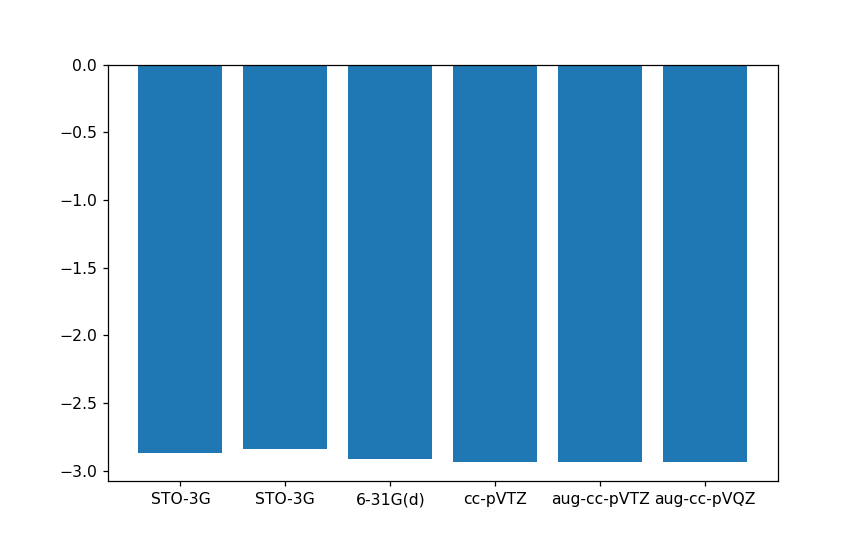Out:
<Container object of 6 artists>

The energy calculated is getting close to the Hartree-Fock limited which is not the real energy of the molecule as it can get even lower if the correlation energy is included. These are the many-body effects which are being ignored in the mean-field approximation to speed up the calculations. The more accurate energy for HeH+ is -2.977537 (CCSD(T) aug-cc-pVQZ). So the mean-field approximation is getting very close to the full energy.

## Molecular orbitals¶

Let's consider the shape of the molecular orbtials and electron density for this molecule along the bond length.

In :
C = np.matmul(X,Cprime)
P = np.array([[ 1.28614168,0.54017322],
[ 0.54017322 ,0.22687011]])

x = np.linspace(-8,8,num=1000)
r1 = abs(x+R/2)
r2 = abs(x-R/2)

psi_CGF_STO3G_He = Coeff[2,0]*(2*Expon[2,0]/np.pi)**(0.75)*np.exp(-Expon[2,0]*r1**2) \
+ Coeff[2,1]*(2*Expon[2,1]/np.pi)**(0.75)*np.exp(-Expon[2,1]*r1**2) \
+ Coeff[2,2]*(2*Expon[2,2]/np.pi)**(0.75)*np.exp(-Expon[2,2]*r1**2)

psi_CGF_STO3G_H = Coeff[2,0]*(2*Expon[2,0]/np.pi)**(0.75)*np.exp(-Expon[2,0]*r2**2) \
+ Coeff[2,1]*(2*Expon[2,1]/np.pi)**(0.75)*np.exp(-Expon[2,1]*r2**2) \
+ Coeff[2,2]*(2*Expon[2,2]/np.pi)**(0.75)*np.exp(-Expon[2,2]*r2**2)

density = np.zeros(x.shape)

density = density + P[0,0]*psi_CGF_STO3G_He*psi_CGF_STO3G_He
density = density + P[1,1]*psi_CGF_STO3G_H*psi_CGF_STO3G_H
density = density + 2*P[0,1]*psi_CGF_STO3G_He*psi_CGF_STO3G_H

In :
plt.figure(figsize=(7,4))
plt.title("Molecular orbitals")

plt.plot(x,C[0,0]*psi_CGF_STO3G_He+C[1,0]*psi_CGF_STO3G_H,label="Bonding")
plt.plot(x,C[0,1]*psi_CGF_STO3G_He+C[1,1]*psi_CGF_STO3G_H,label="Anti-bonding")
plt.legend(loc=4)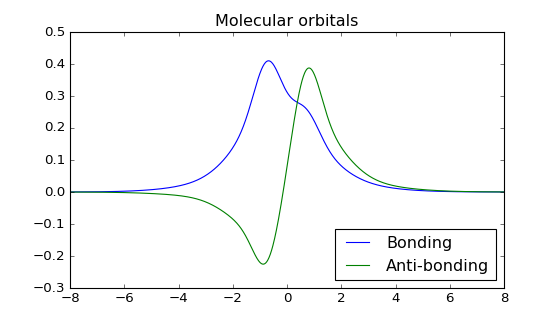Out:
<matplotlib.legend.Legend at 0x111c98400>
In :
plt.figure(figsize=(7,4))
plt.title("Electron density $\Psi^{2}$")
plt.plot(x,density)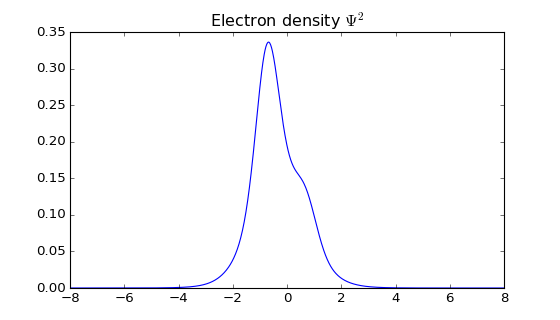Out:
[<matplotlib.lines.Line2D at 0x114e35400>]

Let's compare these results with a larger basis set. This is the input script for the program Gaussian which can use much larger basis sets.

#p RHF/aug-cc-pVQZ SP

HeH+_energy

1 1 He -1.41702 0.00000 0.00000 H -0.82048 0.00000 0.00000

Output energy is -2.932878077558184 which is close to pySCF solution.

Let's plot the wavefunctions and the electron density.

In :
LUMO = np.genfromtxt('LUMOHeH+.txt')
HOMO = np.genfromtxt('HOMOHeH+.txt')
density = np.genfromtxt('densityHeH+.txt')

plt.figure(figsize=(7,4))
plt.title("Molecular orbitals RHF/aug-cc-pVQZ")
plt.plot(-LUMO[750:2250,2]/0.529177*2,LUMO[750:2250,4],label='LUMO')
plt.plot(-HOMO[750:2250,2]/0.529177*2,HOMO[750:2250,4],label='HOMO')
plt.figure(figsize=(7,4))
plt.title("Electron density $e\AA^3$ RHF/aug-cc-pVQZ")
plt.plot(-density[750:2250,2]/0.529177*2,density[750:2250,4],label='density')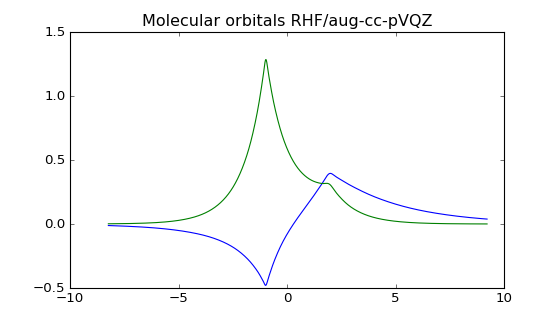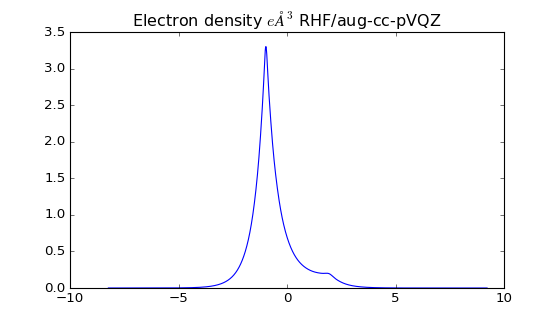Out:
[<matplotlib.lines.Line2D at 0x1162b8198>]

The larger basis set provides more electron density on the Helium atom. You can also see how well the cusp is modelled with many basis sets added together.

If we grab the fchk file we can plot the molecular orbitals in three-dimensions using Avogadro software. Here is the occupied molecular orbital HOMO the surface is the isosurface at the 0.02 density value.

Occupied orbital HOMO

And the unoccupied orbital LUMO

We can also plot the electron density at the 0.002 $e$Å$^3$ value which is roughly where the exchange energy is very strong and you have repuslion between the molecules.

## Conclusions¶

You may be considering that these calculations overly complicated for a simple diatomic such as HHe+ and attempting anything larger would be a nightmare. Fortunately many free publically accessible codes are available for these calculations. If you want to get your hands on something right now there is the website called http://molcalc.org that allows you to do calculations on simple molecules right from your web browser. If you want to do some proper calculations I would recommend getting a copy of the software packages NWChem or GAMESS. To construct your own geometries you will need a program like Avogadro which can be used to build molecules and the input text files you need as input to these quantum chemistry codes.

In :
# Stylings from http://lorenabarba.com
from IPython.core.display import HTML
def css_styling():
return HTML(styles)
css_styling()

Out:

1.Great example, thanks for sharing. To complete it, is there a download link available of the LUMO, HOMO and density input files?

1.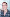Here you go https://www.dropbox.com/s/wtevsqcjr18gmwo/HOMOHeH%2B.zip?dl=0

2.Hello, this post helps me a lot, because you let, in a very clear way the corresponding calculations, thanks a lot.
I have a question, How do you obtain the coefficients for the Gaussian functions?

best regards

1.These are given by the matrix C[]. You can see how I made use of these coefficients to draw the molecular orbitals plt.plot(x,C[0,0]*psi_CGF_STO3G_He+C[1,0]*psi_CGF_STO3G_H,label="Bonding")

3.Hi,
Thanks for your blog. It is really helpful. Can you explain, why the Hartree-Fock explained in Modern Quantum Chemistry by Szabo and Ostlund for HeH+ does not produce the same result as PhSCF(STO-3G). For Zzabo and Ostlund algorithm, the total energy is -2.8606621637 but for PhSCF (STO-3G), it is -2.8418364990824458. What is the difference between these two and what should be changed in Zzabo and Ostlund algorithm so it can produce PhSCf result.

1.I think it has to do with the algorithm used to perform the integration. PySCF uses an advanced library for calculating the integrals see https://onlinelibrary.wiley.com/doi/full/10.1002/jcc.23981 (or preprint https://arxiv.org/pdf/1412.0649.pdf). This example, however, makes use of approximations from the 1970s to solve the integrals (see https://aip.scitation.org/doi/10.1063/1.432807), which would be expected to be less accurate. If you are able to find out anything more about this please let me know!

2.Thanks, Let me see

3.This turns out to be due to Szabo and Ostlund using a different value for Zeta2. This has now been changed to match that of PySCF.

4.Hi,
Thank you for this blog.

I understand that T11 is the kinetic energy operator for the first atom and T22 is the kinetic energy operator for the second atom. But I can't understand why are we calculating T12.

T11 = T11 + T_int(A1[i],A1[j],0.0)*D1[i]*D1[j]
T12 = T12 + T_int(A1[i],A2[j],R2)*D1[i]*D2[j]
T22 = T22 + T_int(A2[i],A2[j],0.0)*D2[i]*D2[j]

1.Thank you for your interest. The diagonal entries of the one-electric kinetic energy operator T11 and T22 are the QM kinetic energy of each electron. The off-diagonal terms T12 describe the quantum mechanical effects of bonding. These terms go to zero at infinity but are non-zero with overlap due to constructive interference. I would recommend the discussion in Szabo and Ostlund textbook section 3.5.2 and the paper https://aip.scitation.org/doi/pdf/10.1063/1.4875735

5.Hello,
Thanks a lot for the great efforts. I have a question and I wish you can help me with. According to you and to Szabo, the calculations of HF on HEH+ gives the same result of the ground state energy of He. Am I right, or there is sth I missed?

1.They are isoelectronic meaning they have the same number of electrons in the same orbitals (s-orbital in this case) however the potential will be different for He and HeH+ and therefore they will have different energies. Using Gaussian 16 at the HF/cc-pVTZ level of theory I got HeH+=-2.932253 and He=-2.8611533

2.Very helpful. Thank you so much.

6.Hi, this is great! Can you provide the original Jupyter file, so that we can play around with it? Cheers!

1.Thanks!

Unfortunately, I am in lockdown in another country without my laptop so can't give you the version on the blog. But I did find an early version of the code in an email that I pushed to github (https://github.com/nzjakemartin/HF/blob/master/HF.ipynb).

I will update this when I get back to my laptop.

Thanks again

2.Thank you! Pls, whenever you are with your laptop, please post the current version here! Saty safe and cheers!

7.Thanks a lot!

8.Very nice post. Just note that if you put the Zeta for He as 1.69, then you will get E = -2.84184 a.u. This Zeta value is from https://www.basissetexchange.org.

1.This comment has been removed by the author.

9.Good job and nice post. A bug is found: G[i,j]=G[i,j]+P[k,l]*(TT[i,j,k,l]-0.5*TT[i,j,k,l]), which should be G[i,j]=G[i,j]+P[k,l]*(TT[i,j,k,l]-0.5*TT[i,l,k,j])

1.This bug change does not change the final SCF energy but it does speed up the SCF loop so thanks for finding this.

10.Zeta is zero in this case so it it not critical to include.